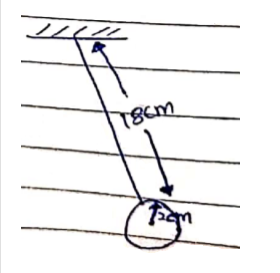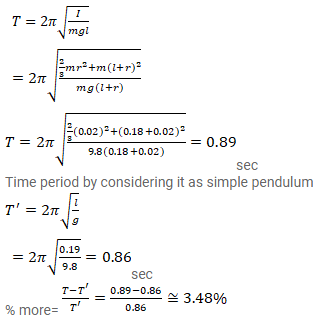# A hollow sphere of radius 2cm is attached to an 18cm

Question:

A hollow sphere of radius $2 \mathrm{~cm}$ is attached to an $18 \mathrm{~cm}$ long thread to make a pendulum. Find the time period of oscillation of this pendulum. How does it differ from the time period calculated using the formula for a simple pendulum?

Solution: